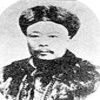#### You may also like### The Mean Game

Edward Wallace based his A Level Statistics Project on The Mean Game. Each picks 2 numbers. The winner is the player who picks a number closest to the mean of all the numbers picked.### Very Old Man

Is the age of this very old man statistically believable?### The Random World

Think that a coin toss is 50-50 heads or tails? Read on to appreciate the ever-changing and random nature of the world in which we live.

# The Derren Brown Coin Flipping Scam

### Why do this problem?

This problem provides a framework for discussion about risk and probability and highlights the stark differences between experimental and theoretical probability.

### Possible approach

You can use this resource at various points in a probability or statistics course. Because the questions aren't simply of the 'calculate this' form, rich disussion should occur, along with various probabilistic computations. It will be very useful to get students thinking about how to formulate questions in statistics and raise many issues concerning chance, averaging and spread.

You might work through many questions in a single lesson or split the questions up and use them as short starters to help get students into statistical frames of mind.

### Key questions

Does the result of a particular flip depend on the results of an previous flips?

### Possible extension

Students can try to invent their own questions based around this activity. They might also consider trying 'Discussing Risk and Reward'.

### Possible support

Students might find the open parts confusing in this problem or they might simply not be ready for the necessary probabilistic calculations. You could re-formulate the questions in a closed 'calculate this' way. Alternatively you could simply suggest that students try to clearly explain what needs to be calculated and discuss how if might be calculated in principle, focussing on which parts are dependent and which parts independent.